## 3D Audio Concepts

SoLoud can perform 3d audio calculations. If you do not need 3d (or "positional") audio, you can skip this chapter.

In practise, all the "3d audio" does is adjust panning and play speed of your audio sources, and as such can be seamlessly used with any "2d audio" that you may also have. This means that background music, for instance, does not need to be represented in the "3d world" in any way.

Any audio source can be 3d, including mixing busses. However, true 3d positioning only really makes sense for mono audio sources.

The doppler and attenuation calculations follow the OpenAL equations.

In order to use the 3d audio, use the 3d versions of the play commands, adjust the positions and velocities of your audio sources and listener with the set3dSource...() and set3dListener...() calls, and call update3dAudio() to ask SoLoud to recalculate the proper panning (and play speed, for doppler).

``````
gSndHandle_orbit = gSoloud.play3d(gSfx_orbit,
50, 0, 0);
// ...
gSoloud.set3dSourceParameters(gSndHandle_orbit,
orbitx, 0, orbitz,
orbitxv, 0, orbitzv);

// ...
gSoloud.update3dAudio();```
```

### Custom Colliders

Sound sources may have a custom collider applied to them. This can be useful in many cases. For instance, if you have a river and want a water flow ambience to play when the player is near the water, you can either have a bunch of audio sources along the river (wasting a lot of voices) or you could have one audio source with a custom collider that checks if the player is near the river and adjusts volume accordingly.

Custom colliders are created by extending the AudioCollider class, which only has one function - collide. The function returns the calculated volume level. Once the custom collider class is made, you can set the collider to an audio source via set3dCollider() call. The call also takes an optional aUserData integer, which can be used to differentiate between the sounds. The same value is provided to the collide() call.

``````
MyCustomCollider cc;
gSound.set3dCollider(&cc);
gSoloud.play(gSound);```
```

The collide() call is made from update3dAudio() before directional panning is calculated, so it is possible to update the positions from inside your collide() function. That way you could figure out the general direction the sound should be coming from (thinking again of the river example), instead of just having a general volume fade.

### Attenuation

Attenuation, or how audio volume decreases on distance, can be calculated in several ways. SoLoud supports three different modes (in addition to "no attenuation"): inverse distance, linear distance and exponential distance. These are calculated using the "clamped" models of OpenAL formulas.

All of the formulas take three parameters: rolloff factor, minimum and maximum distance. How these parameters affect the curves can be seen in the graphs below.

#### Inverse Distance

``````
distance = CLAMP(distance, min_distance, max_distance)
result = min_distance / (min_distance +
rolloff_factor * (distance - min_distance))```
```The higher the rolloff factor, the more steeply the volume drops. At low enough rolloff factor, the volume never drops near zero. Values over 1 recommended (unless you have special needs). Values less than equal to zero result in undefined behavior.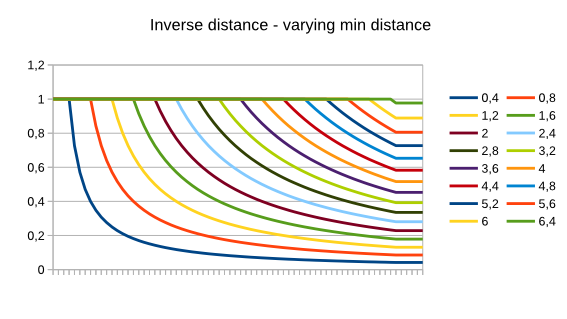Increasing the minimum distance pushes the start of the attenuation further. It also causes the curve to change. Note that the minimum distance must be above 0.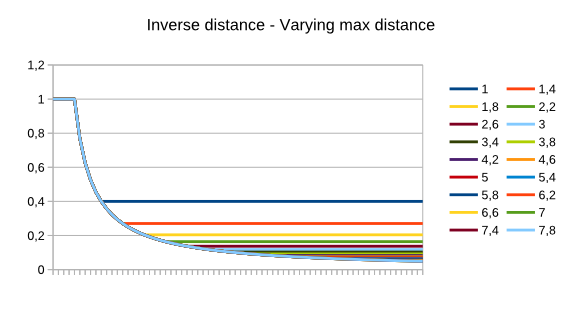The maximum distance simply cuts the attenuation at the volume level it has reached at that point.

#### Linear Distance

``````
distance = CLAMP(distance, min_distance, max_distance)
result = 1 - rolloff_factor *
(distance - min_distance) / (max_distance - min_distance)```
```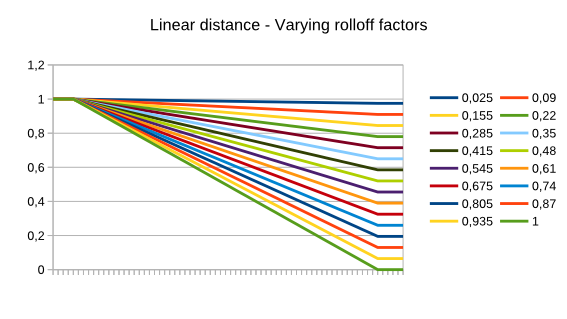The rolloff factor for linear distance simply sets the maximum volume reduction. Using values outside the 0..1 range causes undefined behavior.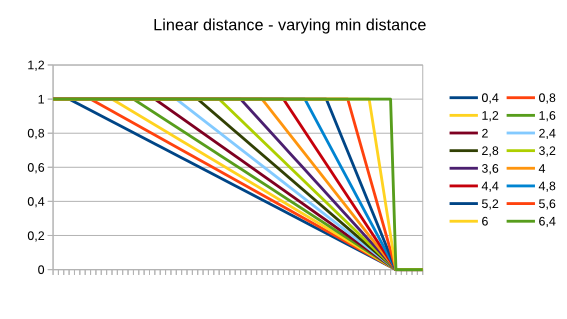The minimum distance works as one might expect. Minimum distance must be less or equal to maximum distance.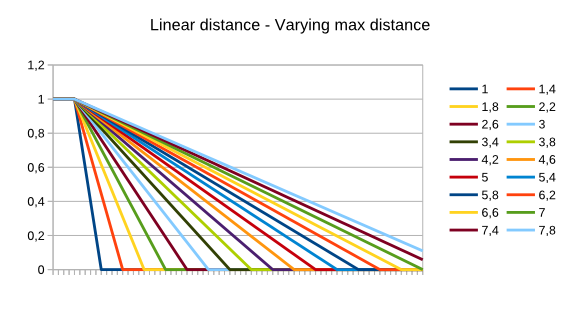The maximum distance works as one might expect. Minimum distance must be less or equal to maximum distance.

#### Exponential Distance

``````
distance = CLAMP(distance, min_distance, max_distance)
result = pow(distance / min_distance, -rolloff_factor)```
```The higher the rolloff factor, the more steeply the volume drops. At low enough rolloff factor, the volume never drops near zero. Values over 1 recommended (unless you have special needs). Values less than equal to zero result in really weird behavior.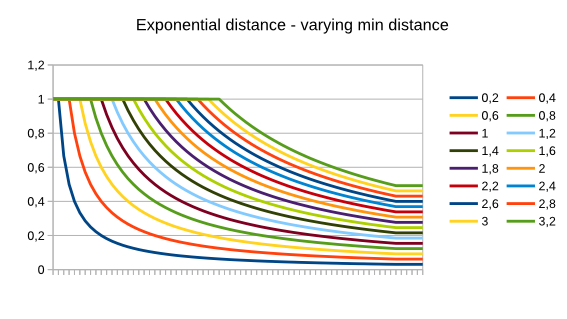Increasing the minimum distance pushes the start of the attenuation further. It also causes the curve to change. Note that the minimum distance must be above 0.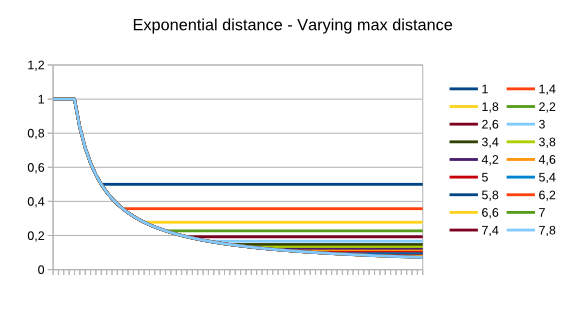The maximum distance simply cuts the attenuation at the volume level it has reached at that point.

### Doppler

"Doppler effect" is the physical phenomenon that causes sound sources (like an ambulance) to sound higher-pitched when they're coming towards you and lower-pitched when going away.A stationary sound (with a stationary listener) receives sound waves as you'd expect. When the sound source (or listener) are moving, the sound waves get "squashed" (for higher-pitch sound) or "stretched" (for lower-pitch sound) depending on whether the sound is approaching or receding from the listener.

SoLoud uses the OpenAL 1.1 formula for doppler calculation. The calculation depends on the listeners' and sound sources' velocities being properly calculated on the application's side. If you do not wish to use the doppler, simply leave all velocities at zero.

In addition to velocities, the doppler depends on the proper value of speed of sound. The default value is set at 343, which assumes that your world coordinates are in meters (where 1 unit is 1 meter), and that the environment is dry air at around 20 degrees Celsius. If those assumptions do not match your environment, change the speed with set3dSoundSpeed().

``````
soloud.set3dSoundSpeed(1497); // we're in water```
```

For a bit of artistic control, you can also set the doppler factor on a per-audio source basis to increase or decrease the strength of the effect. The default value is 1.0.

### Distance Delay

SoLoud can also delay the start of the effects by their distance. This uses the sound speed value and the distance between the listener and the sound source. Since this may be seen as a glitch as most games do not bother simulating this, it is disabled by default. To enable, use the set3dDistanceDelay() function on your sound sources.

``````
snipershot.set3dDistanceDelay(1);```
```

### Speaker Output

Speakers are defined as 3d vectors, and the volume at which each speaker plays is calculated like:

``````
volume = (dot(speaker_vector, sound_vector) + 1) / 2```
```

In practise this ((dot+1)/2) calculation creates a field where sounds that come from the same direction as the speaker play at maximum volume, while sounds that come from exact opposite direction play at zero volume, and anything in between gets a reduced volume.This algorithm is easily applied to any number of speakers in any positioning in 3d space. It may not be as clear-sounding as "Vector Base Amplitude Panning (VBAP)", but it's really easy to implement.